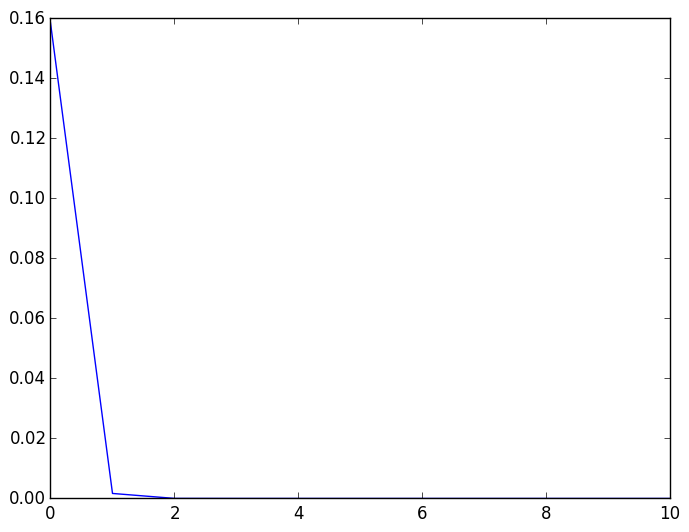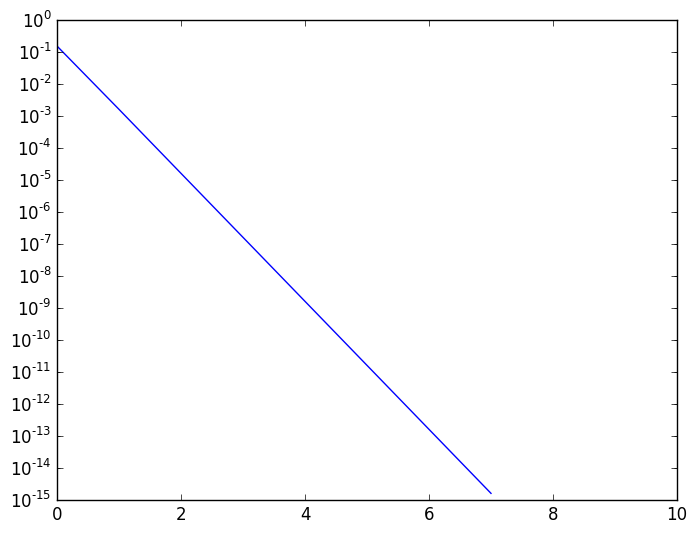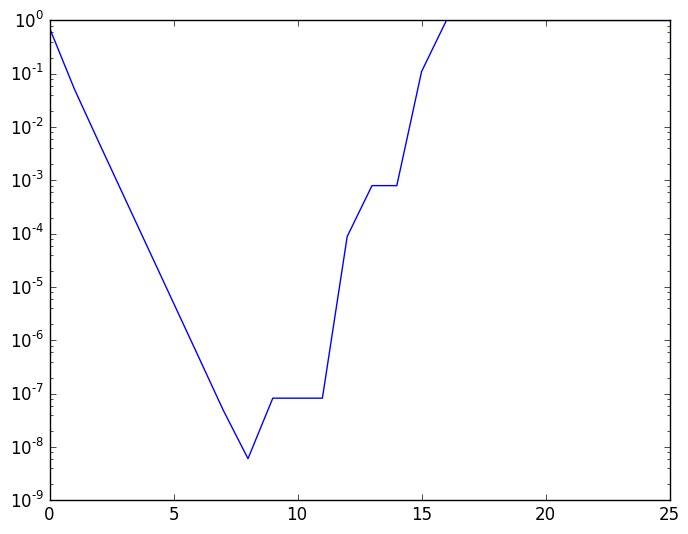# Lecture 5: Rounding and arithmetic in IEEE Floating¶

Before we begin, we need to setup printing floating point numbers in colour.

In :
printred(x)=print("\x1b[31m"*x*"\x1b[0m")
printgreen(x)=print("\x1b[32m"*x*"\x1b[0m")
printblue(x)=print("\x1b[34m"*x*"\x1b[0m")

function printbits(x::Float16)
bts=bits(x)
printred(bts[1:1])
printgreen(bts[2:7])
printblue(bts[8:end])
end

function printbits(x::Float32)
bts=bits(x)
printred(bts[1:1])
printgreen(bts[2:2+8-1])
printblue(bts[2+8:end])
end

function printbits(x::Float64)
bts=bits(x)
printred(bts[1:1])
printgreen(bts[2:2+11-1])
printblue(bts[2+11:end])
end

Out:
printbits (generic function with 3 methods)

Let's start with the example from last lecture. We want to understand how numbers are rounded, by looking at rounding a Float64 to a Float32. Note that putting f0 at the end of a number forces it to be a Float32. Therefore, 1.3f0 is equivalent to calling Float32(1.3).

In :
printbits(1.3) # Float64

0011111111110100110011001100110011001100110011001100110011001101
In :
printbits(1.3f0) #Float32

00111111101001100110011001100110

Note that the last two bits are different. This is because the number has been rounded to the nearest Float32. Because the exponents of both 1.3 and 1.3f0. Both 1.3 and 1.3f0 have the same exponent:

In :
exponent(1.3),exponent(1.3f0)

Out:
(0,0)

We can therefore focus on the significands. The following commands get the significands of the two numbers:

In :
x=1.3
str=bits(1.3)
bts64=str[13:end]  # lets get the bits for the significand.  This uses the
# end keyword for getting all the characters of a string
# up to the last one

Out:
"0100110011001100110011001100110011001100110011001101"
In :
x=1.3
str=bits(Float32(1.3))
bts32=str[10:end]  # lets get the bits for the significand.  This uses the
# end keyword for getting all the characters of a string
# up to the last one

Out:
"01001100110011001100110"

A brief aside: parse interprets a string as a number in base-2, not a sequence of bits. For example, a negative number is given using a minus sign:

In :
parse(Int64,"-11111111110100110011001100110011001100110011001100110011001101",2)

Out:
-4608533498688228557

Let's create a new Float64 by writing bits directly, and see how it is rounded when it becomes a Float32. We begin with the bits of 1.3. The following parses the bts as an unsigned 64-bit integer, then reinterpret the bits as a Float64, creating a Float64 called x64 with the precise bits specified in the original string. We then round it to a Float32 called x32. Checking the bits of x32 shows the result of rounding.

In :
# this is the 32 bit string, aligned so that the significands are in the same column
"00111111101001100110011001100110"
str="0011111111110100110011001100110011001100110011001100110011001101"

bts=parse(UInt64,str,2)
x64=reinterpret(Float64,bts)
x32=Float32(x)
bits(x32)

Out:
"00111111101001100110011001100111"

If we change the first bit after where the significand is truncated to 1, it now rounds up to the nearest Float32:

In :
# this is the old 32 bit string, aligned so that the significands are in the same column
"00111111101001100110011001100110"
str="0011111111110100110011001100110011011100110011001100110011001101"

bts=parse(UInt64,str,2)
x=reinterpret(Float64,bts)
x32=Float32(x)
bits(x32)

Out:
"00111111101001100110011001100111"

We are rounding to the nearest representable Float32. But if all the bits are zero, there is no longer a unique nearest Float32. The default behaviour is to round to the nearest Float32 that has a zero in the last bit:

In :
"00111111101001100110011001100110"
str="0011111111110100110011001100110011010000000000000000000000000000"

bts=parse(UInt64,str,2)
x=reinterpret(Float64,bts)
bits(Float32(x))

Out:
"00111111101001100110011001100110"

The default rounding mode can be changed:

In :
with_rounding(Float32,RoundUp) do
bits(Float32(1.3) )
end

Out:
"00111111101001100110011001100111"
In :
with_rounding(Float32,RoundDown) do
bits(Float32(1.3) )
end

Out:
"00111111101001100110011001100110"
In :
with_rounding(Float32,RoundNearest) do
bits(Float32(1.3) )
end

Out:
"00111111101001100110011001100110"
In :
with_rounding(Float32,RoundToZero) do
bits(Float32(1.3) )
end

Out:
"00111111101001100110011001100110"

A real number can have an infinite number of digits to represent exactly. Define the operation that takes a real number to its Float64 representation as round.

The Arithmetic operations '+', '-', '*', '/' are defined by the property that they are exact up to rounding. That is, if x and y are Float64, we have $$x\oplus y={\rm round}(x+y)$$ where in this formula $\oplus$ denotes the floating point definition of + and + denotes the mathematical definition of +.

This has some bizarre effects. For example, 1.1+0.1 gives a different result than 1.2:

In :
x=1.1
y=0.1
x+y-1.2

Out:
2.220446049250313e-16

This is because ${\rm round}(1.1)\neq1+1/10$, but rather:

$${\rm round}(1.1) = 1 + 2^{-4}+2^{-5} + 2^{-8}+2^{-9}+\cdots + 2^{-48}+2^{-49} + 2^{-51}= {2476979795053773 \over 2251799813685248} = 1.1 +2^{-51} - 2^{-52} - 2^{-53} - 2^{-56} - 2^{-57} - \cdots$$
In :
printbits(1.1)

0011111111110001100110011001100110011001100110011001100110011010
In :
printbits(x)

0011111111110001100110011001100110011001100110011001100110011010
In :
printbits(y)

0011111110111001100110011001100110011001100110011001100110011010
In :
printbits(x+y)

0011111111110011001100110011001100110011001100110011001100110100
In :
printbits(1.2)

0011111111110011001100110011001100110011001100110011001100110011

# Cancellation and calculating derivatives¶

How do I calculate $f'(0)$? The definition $$f'(0) = \lim_{h \rightarrow 0} {f(h) - f(0) \over h}$$ tells us that $$f'(0) \approx {f(h) - f(0) \over h}$$ provided that $h$ is sufficiently small.

Let's try sin(x)

In :
h=0.000001
y=abs(sin(h)./h-1)

Out:
1.66644475996236e-13

We can do a plot to see how fast the error goes down as we let $h$ become small.

Here, we use the notation x:st:y to denote a range of numbers from x to y in steps of size st. So 0:-1:-10 is code for 0,-1,-2,…,-10. Similarly, 1:2:4 is code for 1,3.

We then use 10.0.^(0:-1:-10) to create vector h whose entries are 1,.1,.01,…,1E-10. The .^ syntax is used instead of ^ because, mathematically, a number raised to a vector is not defined. The .^ syntax means perform the operation entrywise.

In :
h=10.0.^(0:-1:-10)

Out:
11-element Array{Float64,1}:
1.0
0.1
0.01
0.001
0.0001
1.0e-5
1.0e-6
1.0e-7
1.0e-8
1.0e-9
1.0e-10

We now use PyPlot to plot the result.

In :
using PyPlot

h=10.0.^(0:-1:-10)
y=abs(sin(h)./h-1)
plot(y);The error decays too fast to interpret the plot. Instead, we use semilogy, which creates a plot whose y-axis is scalled logarithmically.

In :
h=10.0.^(0:-1:-10)
y=abs(sin(h)./h-1)
semilogy(y);So far so good, but now let's try $\exp(x)$:

In :
h=0.0000000000001
(exp(h)-1)/h

Out:
0.9992007221626409

The error is much higher than expected!

In :
h=10.0.^(0:-1:-25)
y=abs((exp(h)-1)./h-1)
semilogy(y);This phenomenon is caused by the effect of rounding when subtracting off two numbers that are close in magnitude, and will be investigated in a future lab.